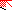# MINLPLib

### A Library of Mixed-Integer and Continuous Nonlinear Programming Instances

#### Instance: ex6_1_3

 Formatsⓘ ams gms mod nl osil Primal Bounds (infeas ≤ 1e-08)ⓘ -0.35249780 p1 ( gdx sol ) (infeas: 6e-17) Other points (infeas > 1e-08)ⓘ Dual Boundsⓘ -0.35249780 (ANTIGONE)-0.35250260 (BARON)-0.35249780 (COUENNE)-0.35249952 (LINDO)-0.35253639 (SCIP) Referencesⓘ Floudas, C A, Pardalos, Panos M, Adjiman, C S, Esposito, W R, Gumus, Zeynep H, Harding, S T, Klepeis, John L, Meyer, Clifford A, and Schweiger, C A, Handbook of Test Problems in Local and Global Optimization, Kluwer Academic Publishers, 1999.McDonald, C M and Floudas, C A, Global Optimization for the Phase and Chemical Equilibrium Problem: Application to the NRTL Equation, Computers and Chemical Engineering, 19:11, 1995, 1111-1139.Castillo, J and Grossmann, I E, Computation of Phase and Chemical Equilibria, Computers and Chemical Engineering, 5:2, 1981, 99-108. Sourceⓘ Test Problem ex6.1.3 of Chapter 6 of Floudas e.a. handbook Added to libraryⓘ 31 Jul 2001 Problem typeⓘ NLP #Variablesⓘ 12 #Binary Variablesⓘ 0 #Integer Variablesⓘ 0 #Nonlinear Variablesⓘ 12 #Nonlinear Binary Variablesⓘ 0 #Nonlinear Integer Variablesⓘ 0 Objective Senseⓘ min Objective typeⓘ nonlinear Objective curvatureⓘ indefinite #Nonzeros in Objectiveⓘ 12 #Nonlinear Nonzeros in Objectiveⓘ 12 #Constraintsⓘ 9 #Linear Constraintsⓘ 3 #Quadratic Constraintsⓘ 6 #Polynomial Constraintsⓘ 0 #Signomial Constraintsⓘ 0 #General Nonlinear Constraintsⓘ 0 Operands in Gen. Nonlin. Functionsⓘ mul log Constraints curvatureⓘ indefinite #Nonzeros in Jacobianⓘ 30 #Nonlinear Nonzeros in Jacobianⓘ 24 #Nonzeros in (Upper-Left) Hessian of Lagrangianⓘ 54 #Nonzeros in Diagonal of Hessian of Lagrangianⓘ 6 #Blocks in Hessian of Lagrangianⓘ 2 Minimal blocksize in Hessian of Lagrangianⓘ 6 Maximal blocksize in Hessian of Lagrangianⓘ 6 Average blocksize in Hessian of Lagrangianⓘ 6.0 #Semicontinuitiesⓘ 0 #Nonlinear Semicontinuitiesⓘ 0 #SOS type 1ⓘ 0 #SOS type 2ⓘ 0 Infeasibility of initial pointⓘ 2.22e-16 Sparsity JacobianⓘSparsity Hessian of Lagrangianⓘ```\$offlisting
*
*  Equation counts
*      Total        E        G        L        N        X        C        B
*         10       10        0        0        0        0        0        0
*
*  Variable counts
*                   x        b        i      s1s      s2s       sc       si
*      Total     cont   binary  integer     sos1     sos2    scont     sint
*         13       13        0        0        0        0        0        0
*  FX      0
*
*  Nonzero counts
*      Total    const       NL      DLL
*         43        7       36        0
*
*  Solve m using NLP minimizing objvar;

Variables  objvar,x2,x3,x4,x5,x6,x7,x8,x9,x10,x11,x12,x13;

Positive Variables  x8,x9,x10,x11,x12,x13;

Equations  e1,e2,e3,e4,e5,e6,e7,e8,e9,e10;

e1.. -((log(x2) - log(x2 + x4 + x6))*x2 + (log(x4) - log(x2 + x4 + x6))*x4 + (
log(x6) - log(x2 + x4 + x6))*x6 + (log(x3) - log(x3 + x5 + x7))*x3 + (log(
x5) - log(x3 + x5 + x7))*x5 + (log(x7) - log(x3 + x5 + x7))*x7 + x2*(
1.44805026165593*x10 + 0.989428667054834*x12) + x4*(1.12676386427658*x8 +
1.00363012835441*x12) + x6*(0.0347225450624344*x8 + 0.82681418300153*x10)
+ x3*(1.44805026165593*x11 + 0.989428667054834*x13) + x5*(
1.12676386427658*x9 + 1.00363012835441*x13) + x7*(0.0347225450624344*x9 +
0.82681418300153*x11)) + objvar =E= 0;

e2.. x8*(x2 + 0.145002897355373*x4 + 0.989528214945409*x6) - x2 =E= 0;

e3.. x9*(x3 + 0.145002897355373*x5 + 0.989528214945409*x7) - x3 =E= 0;

e4.. x10*(0.293701311601799*x2 + x4 + 0.646291923054068*x6) - x4 =E= 0;

e5.. x11*(0.293701311601799*x3 + x5 + 0.646291923054068*x7) - x5 =E= 0;

e6.. x12*(0.619143628558899*x2 + 0.239837817616513*x4 + x6) - x6 =E= 0;

e7.. x13*(0.619143628558899*x3 + 0.239837817616513*x5 + x7) - x7 =E= 0;

e8..    x2 + x3 =E= 0.2995;

e9..    x4 + x5 =E= 0.1998;

e10..    x6 + x7 =E= 0.4994;

* set non-default bounds
x2.lo = 1E-7; x2.up = 0.2995;
x3.lo = 1E-7; x3.up = 0.2995;
x4.lo = 1E-7; x4.up = 0.1998;
x5.lo = 1E-7; x5.up = 0.1998;
x6.lo = 1E-7; x6.up = 0.4994;
x7.lo = 1E-7; x7.up = 0.4994;

* set non-default levels
x2.l = 0.29949;
x3.l = 1E-5;
x4.l = 0.06551;
x5.l = 0.13429;
x6.l = 0.49873;
x7.l = 0.00067;
x8.l = 0.373197867737302;
x9.l = 0.000496390669236887;
x10.l = 0.137685122950498;
x11.l = 0.996764152762375;
x12.l = 0.71260468488485;
x13.l = 0.0203746428730577;

Model m / all /;

m.limrow=0; m.limcol=0;
m.tolproj=0.0;

\$if NOT '%gams.u1%' == '' \$include '%gams.u1%'

\$if not set NLP \$set NLP NLP
Solve m using %NLP% minimizing objvar;

```

Last updated: 2021-03-11 Git hash: 66559cbc